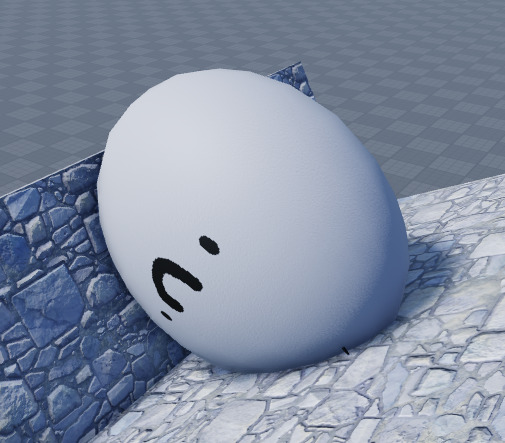# Need a little assistance with a script!

hi,

im making a sort ball game and i need the size to be working, only its not working.
I tried making it a local script a serversided script and even asking friends and people i know.
Nothing worked and no one knew why.

So i have a ball, and that ball size is: 5.75, 5.75, 5.75.
Everytime i weld the ball to the player the ball changes size to match the players size, so instead of being 5.75, 5.75, 5.75 its something else

here are some pics:

normalingame

the script:

``````
local Ball = game.ReplicatedStorage:WaitForChild("Ball"):Clone()

local Weld = Instance.new("Weld")

Weld.Parent = Char

Weld.Part0 = Ball

Weld.Part1 = Char:WaitForChild("HumanoidRootPart")

Ball.Position = Char.PrimaryPart.Position

Ball.Parent = Char
Ball.Size = Vector3.new(5.75, 5.75, 5.75)

local humanoid = Char:WaitForChild("Humanoid")

humanoid.Sit = true

if Char then
for _, part in pairs(Char:GetDescendants()) do
if part:IsA("MeshPart") then
part.Transparency = 1
end
end
end

end)
end)

``````

let me know if you know what the problem is, i really appreciate it.

4 Likes

What is the size of the marble in game? Is the marble a mesh or Roblox’s basepart?

The size of the ball (marble) in game is 4.025, 5.75, 4.888, its a roblox sphere

It thinks its an accessory, don’t ask me why it thinks that, I don’t know
But anyways, try using a WeldConstraint instead of a regular Weld

or try a Motor6D weld

Okay, i will try thanks i will be back in a bit, im online until some what like 23:00

( just tested it and welconstraint didnt do anything )

``````game.Players.PlayerAdded:Connect(function(plr)
local Ball = game.ReplicatedStorage:WaitForChild("Ball"):Clone()
local Weld = Instance.new("Weld")
Weld.Parent = Char
Weld.Part0 = Ball
Weld.Part1 = Char:WaitForChild("HumanoidRootPart")
Ball.Size = Vector3.new(1, 1, 1)
Ball.Position = Char.PrimaryPart.Position
Ball.Parent = Char
Ball.Size = Vector3.new(5.75, 5.75, 5.75)
local humanoid = Char:WaitForChild("Humanoid")
humanoid.Sit = true
if Char then
for _, part in pairs(Char:GetDescendants()) do
if part:IsA("MeshPart") then
part.Transparency = 1
end
end
end
end)
end)

``````

Try this :))

Yo, i tried that but even that does not work, its still egg shaped

can you send the place file? i will try to fix it.

It might be the default scripts are scaling all the character descendants when the character is loaded.
Try parenting the ball to workspace instead.

Parenting it to the workspace works, but it means i have to rewrite the controlls i made

You could try reparenting to the character once the character has fully loaded. I don’t think there is a built in event for this but a simple ‘wait()’ might be just long enough.

How do i do that? Because i tried and i didnt really know how to so i did:

``````		local char = Char:Wait()
``````

and then i did

``````		Ball.Parent = char
``````

and that didnt work

I amended your code slightly, I haven’t checked it fully but added the lines

``````game.Players.PlayerAdded:Connect(function(plr)
local Ball = game.ReplicatedStorage:WaitForChild("Ball"):Clone()

local Weld = Instance.new("Weld")

Weld.Parent = Char

Weld.Part0 = Ball

Weld.Part1 = Char:WaitForChild("HumanoidRootPart")

Ball.Position = Char.PrimaryPart.Position

Ball.Parent = workspace --changed parent to workspace
Ball.Size = Vector3.new(5.75, 5.75, 5.75)

local humanoid = Char:WaitForChild("Humanoid")

humanoid.Sit = true

if Char then
for _, part in pairs(Char:GetDescendants()) do
if part:IsA("MeshPart") then
part.Transparency = 1
end
end
end
Ball.Parent = char --added parent change here
end)
end)``````

that did not work the ball didnt even appear instead it gave:
20:45:30.631 Wait is not a valid member of Model “emiliotigre2017” - Server - Ball:6

what i put on line 6 was

``````local char = Char:Wait()
``````

Weird. Any errors? I made a typo (edited now) due to auto correct.

The error was:
20:45:30.631 Wait is not a valid member of Model “emiliotigre2017” - Server - Ball:6

like i said " what i put on line 6 was"

``````local char = Char:Wait()
``````

Idk if its me or is this really hard to fix or just a dumb over sight and thus a easy fix

That’s not in the code I posted. Try that.

well, in ur code:

so i thought made “char” a thing

Ah sorry my bad, change the letter case to Char ( not char)

What size of the ball when you enter the game? If it not 5.75, 5.75, 5.75 then try this code

``````game.Players.PlayerAdded:Connect(function(plr)
local Ball = game.ReplicatedStorage:WaitForChild("Ball"):Clone()
local Weld = Instance.new("Weld")
Weld.Parent = Char
Weld.Part0 = Ball
Weld.Part1 = Char:WaitForChild("HumanoidRootPart")
Ball.Size = Vector3.new(5.75, 5.75, 5.75)
Ball.Position = Char.PrimaryPart.Position
Ball.Parent = Char

game:GetService('RunService').Heartbeat:Connect(function()
Ball.Size = Vector3.new(5.75, 5.75, 5.75)
end)
end)

local humanoid = Char:WaitForChild("Humanoid")
humanoid.Sit = true
if Char then
for _, part in pairs(Char:GetDescendants()) do
if part:IsA("MeshPart") then
part.Transparency = 1
end
end
end
end)
end)
``````